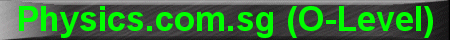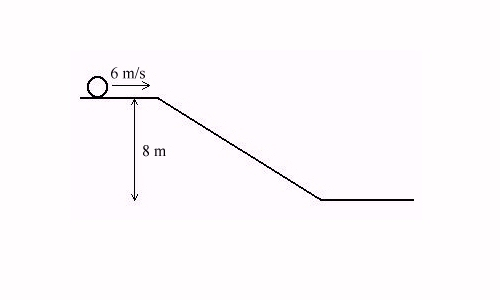(New tips are continually added to these pages.  Check back in a few months' time for more)

TOPIC 4:     Work, Energy and Power

Tip 1:

When applying the Principle of Conservation of Energy, many students like to just apply the equation K.E. = P.E.

Although this may give the correct answer in simple problems, the precise form of the equation should be:

Gain in K.E = Loss in P.E. (for a falling object) ........ (1)

Loss in K.E. = Gain in P.E. (for a rising object) ........ (2)

For example, if an object initially travelling at 6m/s rolls down from the top to the bottom of a frictionless slope, and descends a vertical distance of 8m, what is its velocity at the bottom of the slope?Using equation (1) above:

Gain in K.E = Loss in P.E.

Ĺ m vbottom2 - Ĺ m vtop2 = mgh

Ĺ m vbottom2 - Ĺ m (6)2 = m (10) (8)

Ĺ vbottom2 - Ĺ (6)2 = (10) (8)

vbottom = 14 m s-1